Next Previous Up Contents
Next: Histogram Layer Control
Up: Layer Controls
Previous: Quad Position Layer Control

#### A.4.4.4 Healpix Layer Control

The Healpix layer control (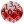) is available from the Sky Plot Window for plotting tables that represent HEALPix maps on the celestial sphere. You can add one of these controls to the stack by using the Add HEALPix Control (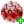) button in the control panel toolbar, or the corresponding item in the Layers menu.

Each row in the table must represent a single healpix tile, and a value from that row is used to colour the corresponding region of the sky plot. The resolution (healpix order) of the input table is supplied or may be guessed in order to do the plot, but the plot may be drawn at a degraded order (bigger pixels) if required.

Note this is different from the SkyDensity form, which takes a table containing sky positions, and represents that as a density map on the healpix grid by gathering the rows up into bins. The control described here works on a table for which that binning has already been done, for instance as a prepared sky map data product, by exporting from a SkyDensity layer, or by executing a suitable (GROUP BY healpix_index) database query.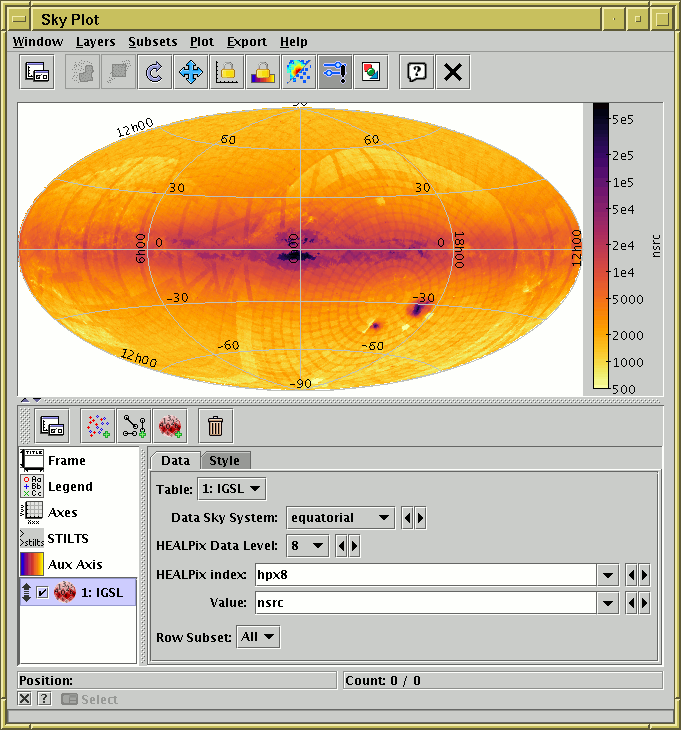Sky Plot Window with a Healpix layer

This control has two tabs, Data and Style.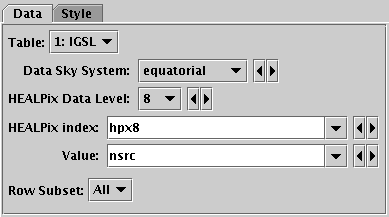Healpix control Data tab

The Data tab lets you specify the table and columns containing the healpix tile data. It has the following fields:

Table
The table supplying the data.
Data Sky System
The sky coordinate system of the grid on which the pixels in the input table are laid out.
HEALPix Data Level
HEALPix level of the (implicit or explicit) tile indices. Values up to 20 are currently supported. This must be the value assumed by the data in the input table. If -1 is selected, an attempt is made to determine the correct level from the data; this may or may not be successful. Using the wrong value here will result in a nonsensical plot, or no plot at all.
HEALPix index
Column in the input table giving HEALPix index value. If this is left blank, then the row index is used, i.e. pixel #0 is assumed to be in the first row etc. This only works if the input table has full sky coverage and the rows are in the correct sequence. Note this value is zero-based, unlike the row index yielded by the special `\$0` or `\$index` token, you would have to write e.g. `\$index-1`.
Value
The column, or other expression, giving the colour to plot for the pixel at each row.
Row Subset
May be used to restrict the plot to one of the defined row subsets.

To control the colour map used to colour the sky tiles, use the Auxfixed control.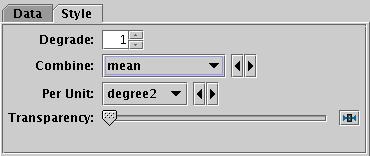Healpix control Style tab

The Style tab lets you configure additional details of the plot's appearance. It has the following fields:

Degrade
Controls the resolution at which the pixel grid is actually plotted. If the value is zero, then the grid plotted is the same as that of the input data, but if a positive value is supplied then the healpix order will be reduced by that many. Each increment means that 4 pixels from the previous order will be combined into one. The way that combination is done is controlled by the Combine option.
Combine
Defines how values degraded to a lower HEALPix level are combined together to produce the value assigned to the larger tile, and hence its colour. This is mostly useful in the case that `degrade`>0.

For density-like values (`count-per-unit`, `sum-per-unit`) the scaling is additionally influenced by the Per Unit option.

The available options are:

• `sum`: the sum of all the combined values per bin
• `sum-per-unit`: the sum of all the combined values per unit of bin size
• `count`: the number of non-blank values per bin (weight is ignored)
• `count-per-unit`: the number of non-blank values per unit of bin size (weight is ignored)
• `mean`: the mean of the combined values
• `median`: the median of the combined values (may be slow)
• `min`: the minimum of all the combined values
• `max`: the maximum of all the combined values
• `stdev`: the sample standard deviation of the combined values
• `hit`: 1 if any values present, NaN otherwise (weight is ignored)

Per Unit
Defines the unit of sky area used for scaling density-like Combine values (e.g. `count-per-unit` or `sum-per-unit`). If the Combination mode is calculating values per unit area, this configures the area scale in question. For non-density-like combination modes (e.g. `sum` or `mean`) it has no effect.

The available options are:

• `steradian`: steradian
• `degree2`: square degree
• `arcmin2`: square arcminute
• `arcsec2`: square arcsecond
• `mas2`: square milliarcsec
• `uas2`: square microarcsec

Transparency
Adjusts the transparency of the filled area.

Next Previous Up Contents
Next: Histogram Layer Control
Up: Layer Controls
Previous: Quad Position Layer Control

TOPCAT - Tool for OPerations on Catalogues And Tables
Starlink User Note253
TOPCAT web page: http://www.starlink.ac.uk/topcat/
Author email: m.b.taylor@bristol.ac.uk
Mailing list: topcat-user@jiscmail.ac.uk